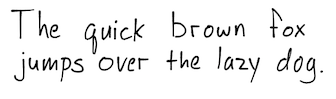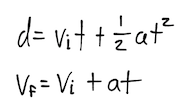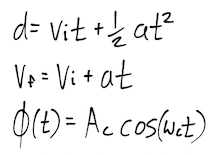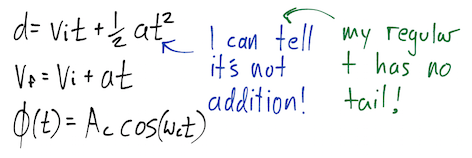# Handwriting

This is my handwriting:I’ve come to realize that my regular handwriting differs from the handwriting that I use when I’m doing math. When using certain letters as variables or symbols in a mathematical context, I write them in a completely different manner than how I write them in sentences. Interestingly (or at least interestingly to me) I can remember precisely why I write these letters differently when they’re in equations. The course of actions that leads to this is always as follows:

1. I learn a new area of mathematics in which I’m required to use a new set of variables.
2. I write the variable using my normal handwriting.
3. I realize that using my current handwriting, I’m unable to differentiate between two distinct symbols. As a result, the math becomes very difficult.
4. I force myself to adopt a new way to write one of the characters that allows me to distinguish between the two.

The first time this happened was when I began to take physics in high school. The began by covering kinematics, and I was introduced to four lovely linear motion equations:

For those unfamiliar with these equations, they describe an object’s motion through a single dimension as a function of time. $t$ is the amount of time that has elapsed, $v_{i}$ is the initial speed, $v_{f}$ is the final speed, $d$ is the object’s displacement, and $a$ is a constant acceleration experienced by that object.

I used to write $t$ as follows:Hastily written, the $t$ looks very much like an addition sign. This was never a problem, as up to this point I simply avoided using $t$ as a variable when doing math. Unfortunately, in my new physics class, this was no longer viable.

For a while, I continued to write $t$ as I had previously done. I distinctly remember a quiz where I got so jumbled up between my addition signs and my time variable that I erased my entire solution to start fresh, only to have the quiz period end immediately after doing so.

Displeased with my glorious quiz grade of 0%, I started writing $t$ with a little tail at the bottom, which easily differentiated it from an addition sign. Since then, I’ve always written $t$ in this manner when doing mathematics:Peculiarly, I still omit the tail when I’m not doing math.